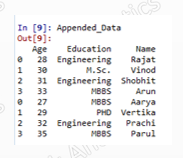## Pages

###Python Tutorial 5.0

Appending, which means bring pieces of similar information together or make a vertical stack of data.

Often for no reasons, people get confused between merging and appending. Here, once again, we would try to make it crystal clear with visual illustration.

Let's learn how appending is performed in Python!

### How is appending different from merging?

Appending is generally used when various pieces of same information has to be collated together. e.g. Monthly Sales data, monthly premium data etc.

Merging on the other hand is used when different information pieces for the same entity are to be collated together. It requires a primary common key in the datasets to be merged.

### Appending in Python

#Let's first create two data with list of speakers at a conference:

import pandas as p

List1_Dictionary= {
'Name'  : ['Rajat','Vinod','Shobhit','Arun'],
'Age'     : [28,30,31,33],
'Education' : ['Engineering','M.Sc.','Engineering','MBBS'] }

List_1=p.DataFrame(List1_Dictionary, columns=['Name','Age','Education'])List_1
List_1

List_2_data = {
'Age'       :   [27,29,32,35],
'Name'      :   ['Aarya','Vertika','Prachi','Parul'],
'Education' :   ['MBBS','PHD','Engineering','MBBS'] }

List_2=p.DataFrame(List_2_data,columns=['Age','Name','Education'])

List_2List_2

# Let's now append the lists - simply with concat functionAppended_Data

Appended_Data=p.concat([List_1,List_2])

Appended_Data

The above example was very basic one as both were having same columns so concat function simply placed one data below other.

Important point : The order of the variables in the data is not mandatory to be same for appending. Resultant dataset, though, maintains the column in ascending order.

### # Complication 1 : Inconsistent column names

# Suppose in the above example, if we replace 'Name' column with 'Student_Name' in List_1 dataset then the output would be

Python doesn't  throw an error but will give above output, which ideally should not be the output and is quite unusual. In order to avoid such situation we need to rename the column to make column names uniform. Hence let's rename columns.

List_1=List_1.rename(columns = {'Student_Name':'Name'})

After renaming the column you would get the same output of "Appended_Data"(Given in first example).

Complication 2 : Different columns in datasets

We have additional columns in the first data set.

List1_Dictionary= {
'Name'  : ['Rajat','Vinod','Shobhit','Arun'],
'Age'     : [28,30,31,33],
'Education' : ['Engineering','M.Sc.','Engineering','MBBS'],
'City' : ['New Delhi', 'Mumbai', 'Bangalore', 'Calcutta']
}

List_1=p.DataFrame(List1_Dictionary, columns=['Name','Age','Education','City'])

List_1

List_2_Dictionary = {
'Age'       :   [27,29,32,35],
'Name'      :   ['Aarya','Vertika','Prachi','Parul'],
'Education' :   ['MBBS','PHD','Engineering','MBBS'] }

List_2=p.DataFrame(List_2_
Dictionary,columns=['Age','Name','Education'])

List_2

Now, let's try appending these datasets

Appended_Data=p.concat([List_1,List_2])

the output will be :

Which is acceptable ... and we are now good with it!

Enjoy reading our other articles and stay tuned with us.

Kindly do provide your feedback in the 'Comments' Section and share as much as possible.